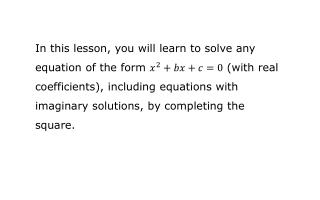DownloadDownload Presentationr eview: completing the square

# r eview: completing the square

Download Presentation## r eview: completing the square

- - - - - - - - - - - - - - - - - - - - - - - - - - - E N D - - - - - - - - - - - - - - - - - - - - - - - - - - -
##### Presentation Transcript

1. In this lesson, you will learn to solve any equation of the form (with real coefficients), including equations with imaginary solutions, by completing the square.

2. review: completing the square

3. review: completing the square Solve . Move away the constant term.

4. review: completing the square Solve . Move away the constant term.

5. review: completing the square Solve . Move away the constant term.

6. review: completing the square Solve . Move away the constant term. Add a number to both sides to complete the square.

7. review: completing the square Solve . Move away the constant term. Add a number to both sides to complete the square.

8. review: completing the square Solve . Move away the constant term. Add a number to both sides to complete the square.

9. review: completing the square Solve . Move away the constant term. Add a number to both sides to complete the square.

10. review: completing the square Solve . Move away the constant term. Add a number to both sides to complete the square.

11. review: completing the square Solve . Move away the constant term. Add a number to both sides to complete the square.

12. review: completing the square Solve . Move away the constant term. Add a number to both sides to complete the square. Take 8. Divide by 2. Square.

13. review: completing the square Solve . Move away the constant term. Add a number to both sides to complete the square. Take 8. Divide by 2. Square.

14. review: completing the square Solve . Move away the constant term. Add a number to both sides to complete the square. Take 8. Divide by 2. Square.

15. review: completing the square Solve . Move away the constant term. Add a number to both sides to complete the square. Factor, square root, and isolate . Take 8. Divide by 2. Square.

16. review: completing the square Solve . Move away the constant term. Add a number to both sides to complete the square. Factor, square root, and isolate . Take 8. Divide by 2. Square.

17. review: completing the square Solve . Move away the constant term. Add a number to both sides to complete the square. Factor, square root, and isolate . Take 8. Divide by 2. Square.

18. review: completing the square Solve . Move away the constant term. Add a number to both sides to complete the square. Factor, square root, and isolate . Take 8. Divide by 2. Square.

19. review: completing the square Solve . Move away the constant term. Add a number to both sides to complete the square. Factor, square root, and isolate . Take 8. Divide by 2. Square.

20. review: writing the square root of a negative number in terms of Simplify .

21. review: writing the square root of a negative number in terms of Simplify .

22. review: writing the square root of a negative number in terms of Simplify .

23. review: writing the square root of a negative number in terms of Simplify .

24. review: writing the square root of a negative number in terms of Simplify .

25. review: writing the square root of a negative number in terms of Simplify .

26. review: writing the square root of a negative number in terms of Simplify .

27. review: writing the square root of a negative number in terms of Simplify .

28. review: writing the square root of a negative number in terms of Simplify .

29. review: writing the square root of a negative number in terms of Simplify .

30. review: writing the square root of a negative number in terms of Simplify .

31. using completing the square with imaginary solutions

32. using completing the square with imaginary solutions Solve . Move away the constant term. Add a number to both sides to complete the square. Factor, square root, and isolate . Take 8. Divide by 2. Square.

33. using completing the square with imaginary solutions Solve . Move away the constant term. Add a number to both sides to complete the square. Factor, square root, and isolate . Take 8. Divide by 2. Square.

34. using completing the square with imaginary solutions: an example Solve .

35. using completing the square with imaginary solutions: an example Solve .

36. using completing the square with imaginary solutions: an example Solve .

37. using completing the square with imaginary solutions: an example Solve .

38. using completing the square with imaginary solutions: an example Solve .

39. using completing the square with imaginary solutions: an example Solve .

40. using completing the square with imaginary solutions: an example Solve .

41. using completing the square with imaginary solutions: an example Solve .

42. using completing the square with imaginary solutions: an example Solve .

43. using completing the square with imaginary solutions: an example Solve .

44. using completing the square with imaginary solutions: an example Solve .

45. using completing the square with imaginary solutions: an example Solve .

46. using completing the square with imaginary solutions: an example Solve .

47. using completing the square with imaginary solutions: an example Solve .